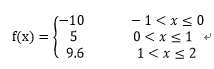# Python OJ 从入门到入门基础练习 10 题

1、天天向上的力量： 一年365天，以第1天的能力值为基数，记为1.0。当好好学习时，能力值相比前一天提高N‰；当没有学习时，由于遗忘等原因能力值相比前一天下降N‰。每天努力或放任，一年下来的能力值相差多少呢？其中，N的取值范围是1到10，N可以是小数。

N = eval(input())

if N==10:
dayup = pow((1.0 + (N / 1000)), 365)
daydown = pow((1.0 - (N / 1000)), 365)
print("{:.2f},{:.2f},{:.0f}".format(dayup, daydown, dayup / daydown))
elif N==5:
dayup = pow((1.0 + (N / 1000)), 365)
daydown = pow((1.0 - (N / 1000)), 365)
print("{:.2f},{:.2f},{:.0f}".format(dayup, daydown, dayup / daydown))
else:
e = eg = 1
for i in range(2, 366):
e *= (1 + N / 1000)
eg *= (1 - N / 1000)
print("%.2f,%.2f,%d" % (e, eg, e / eg))


2、快乐的数字： 编写一个算法来确定一个数字是否“快乐”。 快乐的数字按照如下方式确定：从一个正整数开始，用其每位数的平方之和取代该数，并重复这个过程，直到最后数字要么收敛等于1且一直等于1，要么将无休止地循环下去且最终不会收敛等于1。能够最终收敛等于1的数就是快乐的数字。

• 12 + 92 = 82
• 82 + 22 = 68
• 62 + 82 = 100
• 12 + 02 + 02 = 1

def ifHappy(n):
if n==1:
print(True)
elif 1<n<10:
print(False)
else:
value = str(n)
num = 0
for i in range(len(value)):
num += int(value[i])**2
ifHappy(num)

N = eval(input())
ifHappy(N)


3、跳台阶： 一只青蛙一次可以跳上1级台阶，也可以跳上2级。请问该青蛙跳上一个n级的台阶总共有多少种跳法。输入台阶数，输出一共有多少种跳法。

def fun(a):
total=0
firstElem=1
secondElem=2

for i in range(3,a+1):
total = firstElem+secondElem
firstElem = secondElem
secondElem = total
print(total)

n = eval(input())
fun(n)


4、百分制成绩转换五分制(循环)： 编写一个学生成绩转换程序，用户输入百分制的学生成绩，成绩大于或等于90且小于或等于100的输出为“A”，成绩大于或等于80且小于90的输出为“B”，成绩大于或等于70且小于80的输出为“C”，成绩大于或等于60且小于70的输出为“D”，成绩小于60的输出为“E”。输入数据不合法时输出“data error!”用户可反复输入成绩进行转换，输入负数时输出“end”并结束程序。

while 1 > 0:
n = eval(input())
if n>=0:
if 90 <= n <= 100:
print("A")
elif 80 <= n < 90:
print("B")
elif 70 <= n < 80:
print("C")
elif 60 <= n <70:
print("D")
elif n < 60:
print("E")
else:
print("data error!")
else:
print("end")
break


5、质数判断： 质数(Prime Number)又称素数。一个大于1的自然数，除了1和它自身外，不能被其他自然数整除的数叫做质数；否则称为合数。本题要求实现一个函数，判断参数是否是质数，是的话返回True，否则返回False。

import math

# 定义isPrime函数
def isPrime(a):
if a == 2:
return True
elif a == 3:
return True
else:
for i in range(2,int(math.sqrt(a))+1):
if a%i == 0:
return False
else:
return True

num = int(input())  # 读入并转换为整数类型

if isPrime(num):  # 调用isPrime函数判断num是否为素数
print('yes')
else:
print('no')


6、月份缩写： 如果有 months = "Jan.Feb.Mar.Apr.May.Jun.Jul.Aug.Sep.Oct.Nov.Dec."，编写一个程序，用户输入一个月份的数字，输出月份的缩写。

months = "Jan.Feb.Mar.Apr.May.Jun.Jul.Aug.Sep.Oct.Nov.Dec."
n = input()

#（每个月份的数字-1）*4就是这个月份简写的开始索引，截取4个字符
index = (int(n)-1)*4
month = months[index: index + 4]
print(month)


7、分段函数：N = eval(input())

if -1 < N <= 0:
print("f(x)=-10")
elif 0 < N <= 1:
print("f(x)=5")
elif 1 < N <= 2:
print("f(x)=9.6")
else:
print("ERROR")


8、今年多少天： 闰年366天，其他年份365天。普通年（不能被100整除的年份）能被4整除的为闰年。（如2004年就是闰年,1999年不是闰年）；世纪年（能被100整除的年份）能被400整除的是闰年。(如2000年是闰年，1900年不是闰年)；用户输入一个正整数，代表年份，输出该年有多少天？

N = int(input())

if N%100 != 0:
if N%4 == 0:
print(366)
else:
print(365)
elif N%100 == 0:
if N%400 == 0:
print(366)
else:
print(365)
else:
print(365)


9、验证码较验： 用户登录网站经常需要输入验证码，验证码包含大小写字母和数字，随机出现。用户输入验证码时不区分大小写，只要各字符出现顺序正确即可通过验证。请写一个程序完成验证码的匹配验证，假设当前显示的验证码是'Qs2X'。如果用户输入验证码正确，输出“验证码正确”，输入错误时输出“验证码错误，请重新输入”。

s = "Qs2X"
N = input()
if s.lower() == N.lower():
print("验证码正确")
else:
print("验证码错误，请重新输入")


10、奇数数列求和： 求1+3+5+……+（2n-1）前n项和。

N = eval(input())
sum = 1
for i in range(2,2*N):
if i%2 == 0:
continue
else:
sum += i
print(sum)